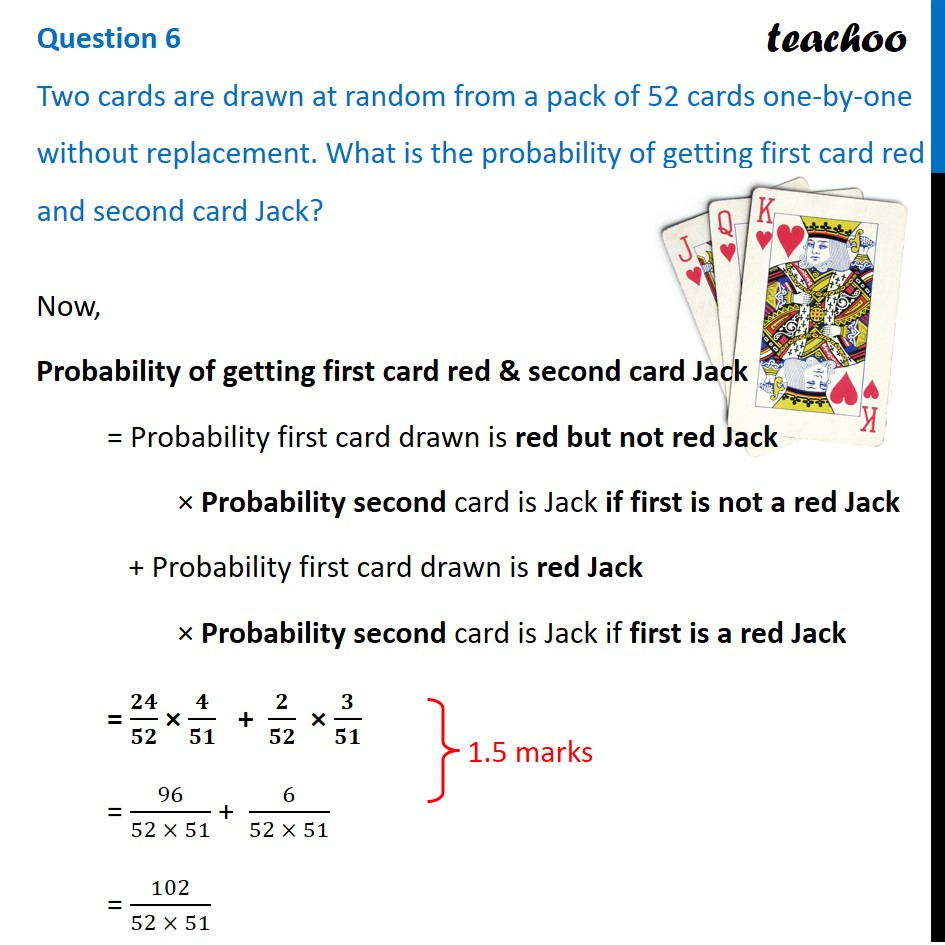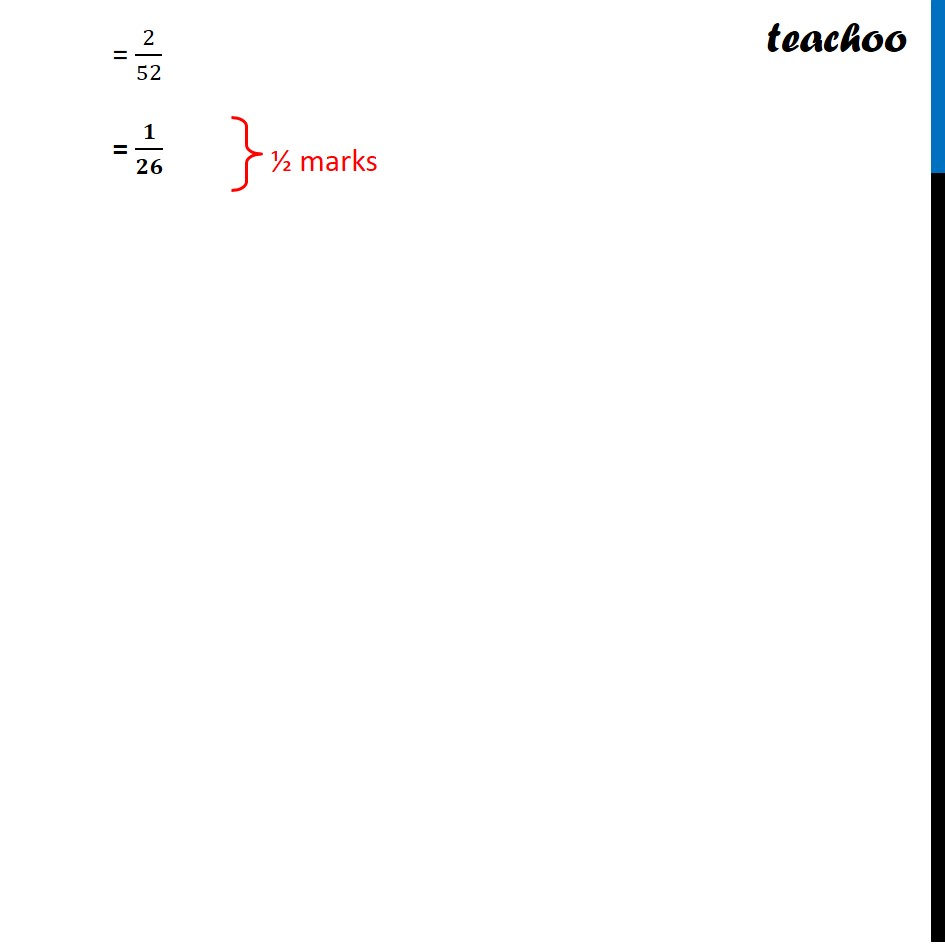CBSE Class 12 Sample Paper for 2022 Boards (For Term 2)

Class 12
Solutions of Sample Papers and Past Year Papers - for Class 12 Boards

## Two cards are drawn at random from a pack of 52 cards one-by-one without replacement. What is the probability of getting first card red and second card Jack?Learn in your speed, with individual attention - Teachoo Maths 1-on-1 Class

### Transcript

Question 6 Two cards are drawn at random from a pack of 52 cards one-by-one without replacement. What is the probability of getting first card red and second card Jack? Now, Probability of getting first card red & second card Jack = Probability first card drawn is red but not red Jack × Probability second card is Jack if first is not a red Jack + Probability first card drawn is red Jack × Probability second card is Jack if first is a red Jack = 𝟐𝟒/𝟓𝟐 × 𝟒/𝟓𝟏 + 𝟐/𝟓𝟐 × 𝟑/𝟓𝟏 = 96/(52 × 51) + 6/(52 × 51) = 102/(52 × 51) = 2/52 = 𝟏/𝟐𝟔